previous lesson

Dispersion Standard Deviation

A Interval data: Standard deviation (abbreviated S when used for a sample)

1. ungrouped data: S =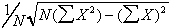Where: x = variable

N = total # in sample

(Caution: x2 means to square all the x's, then sum them up)

Example:

 # of siblings x2 0 0 0 0 0 0 0 0 1 1 1 1 1 1 2 4 2 4 2 4 3 9 4 16 16 =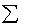x 40=x2

Q: What is the spread or variation in number of siblings?

A: s =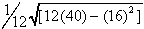s =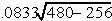=s = .0833(14.97) = 1.25

2. grouped data: s =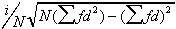Where: i = width of category

f = frequency

N = total cases in sample (or f )

d = step deviations from an arbitrary starting point

* In this class, all "d's" will appear the same.

Example:

 # of siblings f d fd d2 fd2 0 4 0 0 0 0 1 3 1 3 1 3 2 3 2 6 4 12 3 1 3 3 9 9 4 1 4 4 16 16 i = 1 12 = N 16 =fd 40 =fd2

Q: What is the spread or variation in the number of siblings?

A:

S =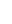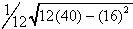S =1.25

STANDARDIZED SCORES: Z-SCORES =# of siblings f fm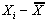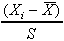0 4 0 0 - 1.333 -1.333/1.25 -1.07 1 3 3 1 - 1.333 -.333/1.25 -0.27 2 3 6 2 - 1.333 .667/1.25 0.53 3 1 3 3 - 1.333 1.667/1.25 1.33 4 1 4 4 - 1.333 2.667/1.25 2.13 12 16

* This example assumes normal distribution even though it is not normally distributed.

Z-SCORE CHART (selected Z-scores)

 Z-Scores Area between the mean and the score Area beyond the mean and the score .27 0.1064 0.3939 .53 0.2019 0.2981 1.00 0.3413 0.1587 1.07 0.3577 0.1423 1.33 0.4082 0.0918 2.00 0.4772 0.228 2.13 0.4834 0.0166 3.00 0.4986 0.0014

NORMAL DISTRIBUTION

+ 1 s will include 68.26% of the cases

+ 2 s will include 95.44% of the cases

+ 3 s will include 99.72% of the cases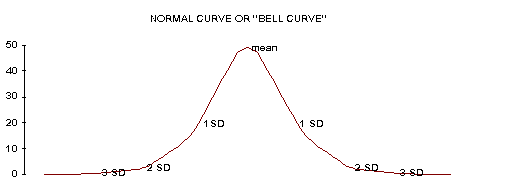so plus or minus 1 SD = 68.26% of the population and 2SD = 95.44% and 3SD = 99.72%

Example: Bank balance (Mr. Jones):

x = \$2000.

s = 500 and distribution = normal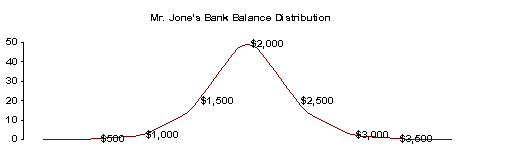Interpretation:

68% of the time, Mr. Jones's balance is between \$1500 - \$2500.

95% of the time, " " " " \$1000 - \$3000.

99.7% of the time, " " " " \$500 - \$3500.

Example: Intelligence Quotient Scores

x = 100 S = 16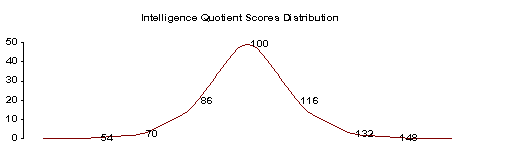Interpretation:

68% of the sample's IQs fall between 86 and 116.

95% of the sample's IQs fall between 70 and 132

99.7% of the sample's IQs fall between 54 and 148

SMOOTH, UNIMODAL (BUT SKEWED) DISTRIBUTION

+ 1s will include 56% of the cases, or more.

+ 2s will include 89% of the cases, or more.

+ 3s will include 95% of the cases, or more.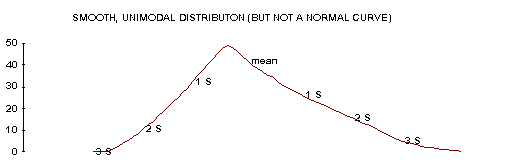Example: Class Averages from SOC201

x = 72.76%

S = 15.48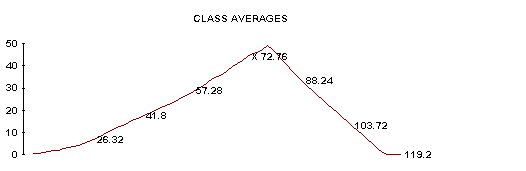Interpretation:

56% or more of the class will average between 57.28 and 88.24%.

89% or more of the class will average between 41.8 and 103.72%

95% or more of the class will average between 26.32 and 119.2%

* It is impossible to average more than 110%, in most classes 100%. In such cases, reword the statements to reflect this, i.e. "26.32 and 110%"

ANY SHAPED DISTRIBUTION (irregular): use this if the distribution of the actual raw data is completely unknown. Notice it has no interpretation for + 1s.

+ 2s will include 75% of the cases, or more.

+ 3s will include 89% of the cases, or more.

Example: Hours Soc201 students are employed per week

x = 21.45 Distribution irregular

S = 9.63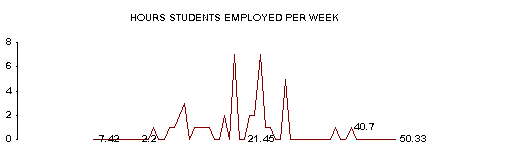Interpretation:

75% or more of the students are employed between 2.2 and 40.7 hrs. a week.

89% or more of the students are employed between 0 and 50.33 hours a week.*

*It is impossible to be employed less than 0 hours per week.

Example: GPA's in Soc201 classes

x = 2.7

S = .4 distribution = irregular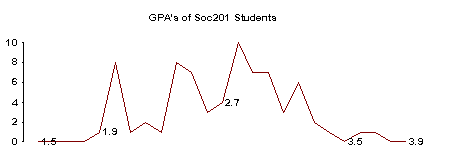Interpretation:

75% or more of the Soc201 students have GPA's between 1.9 and 3.5

89% or more of the Soc201 students have GPA's between 1.5 and 3.9

Example: Frequency Distribution

 # of siblings f 0 4 1 3 2 3 3 1 4 1 12 = N

Q: S = 1.25 in the above frequency distribution, what does this mean?

A: 1) compute the mean: x = 1.33

2) identify the shape of distribution (make a

frequency polygon)

3) interpret the dataInterpretation:

75% or more of the sample have between 0 and 3.83 siblings.*

89% or more of the sample have between 0 and 5.08 siblings.*

*Impossible to have less than 0 siblings